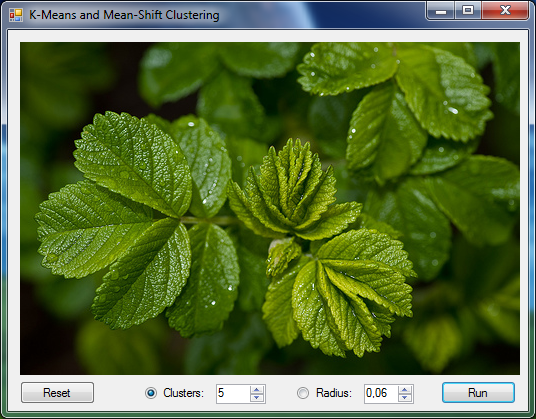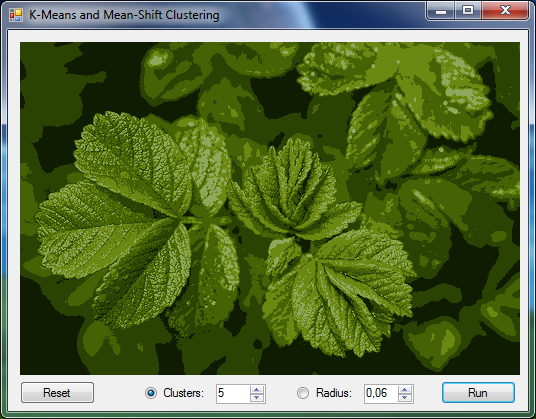﻿ KMeans Class# KMeans Class

Lloyd's k-Means clustering algorithm.Inheritance Hierarchy

Namespace:  Accord.MachineLearning
Assembly:  Accord.MachineLearning (in Accord.MachineLearning.dll) Version: 3.8.0Syntax
```[SerializableAttribute]
public class KMeans : ParallelLearningBase, IUnsupervisedLearning<KMeansClusterCollection, double[], int>,
IClusteringAlgorithm<double[], double>, IClusteringAlgorithm<double[]>,
IUnsupervisedLearning<IClusterCollection<double[]>, double[], int>```

The KMeans type exposes the following members.Constructors
NameDescriptionKMeans(Int32)
Initializes a new instance of the K-Means algorithmKMeans(Int32, IDistanceDouble)
Initializes a new instance of the KMeans algorithmKMeans(Int32, FuncDouble, Double, Double) Obsolete.
Initializes a new instance of KMeans algorithm
TopProperties
NameDescriptionCentroids
Gets or sets the cluster centroids.Clusters
Gets the clusters found by K-means.ComputeCovariances
Gets or sets whether covariance matrices for the clusters should be computed at the end of an iteration. Default is true.ComputeError
Gets or sets whether the clustering distortion error (the average distance between all data points and the cluster centroids) should be computed at the end of the algorithm. The result will be stored in Error. Default is true.Dimension
Gets the dimensionality of the data space.Distance
Gets or sets the distance function used as a distance metric between data points.Error
Gets the cluster distortion error after the last call to this class' Compute methods.Iterations
Gets the number of iterations performed in the last call to this class' Compute methods.K
Gets the number of clusters.MaxIterations
Gets or sets the maximum number of iterations to be performed by the method. If set to zero, no iteration limit will be imposed. Default is 0.ParallelOptions
Gets or sets the parallelization options for this algorithm.
(Inherited from ParallelLearningBase.)Token
Gets or sets a cancellation token that can be used to cancel the algorithm while it is running.
(Inherited from ParallelLearningBase.)Tolerance
Gets or sets the relative convergence threshold for stopping the algorithm. Default is 1e-5.UseSeeding
Gets or sets the strategy used to initialize the centroids of the clustering algorithm. Default is KMeansPlusPlus.
TopMethods
NameDescriptionCompute(Double) Obsolete.
Divides the input data into K clusters.Compute(Double, Double) Obsolete.
Divides the input data into K clusters.Compute(Double, Double) Obsolete.
Divides the input data into K clusters.ComputeInformation(Double)
Computes the information about each cluster (covariance, proportions and error).ComputeInformation(Double, Int32)
Computes the information about each cluster (covariance, proportions and error).converged
Determines if the algorithm has converged by comparing the centroids between two consecutive iterations.Equals
Determines whether the specified object is equal to the current object.
(Inherited from Object.)Finalize
Allows an object to try to free resources and perform other cleanup operations before it is reclaimed by garbage collection.
(Inherited from Object.)GetHashCode
Serves as the default hash function.
(Inherited from Object.)GetType
Gets the Type of the current instance.
(Inherited from Object.)Learn
Learns a model that can map the given inputs to the desired outputs.MemberwiseClone
Creates a shallow copy of the current Object.
(Inherited from Object.)Randomize
Randomizes the clusters inside a dataset.ToString
Returns a string that represents the current object.
(Inherited from Object.)
TopExtension Methods
NameDescriptionHasMethod
Checks whether an object implements a method with the given name.
(Defined by ExtensionMethods.)IsEqual
Compares two objects for equality, performing an elementwise comparison if the elements are vectors or matrices.
(Defined by Matrix.)To(Type)Overloaded.
Converts an object into another type, irrespective of whether the conversion can be done at compile time or not. This can be used to convert generic types to numeric types during runtime.
(Defined by ExtensionMethods.)ToTOverloaded.
Converts an object into another type, irrespective of whether the conversion can be done at compile time or not. This can be used to convert generic types to numeric types during runtime.
(Defined by ExtensionMethods.)
TopRemarks

In statistics and machine learning, k-means clustering is a method of cluster analysis which aims to partition n observations into k clusters in which each observation belongs to the cluster with the nearest mean.

It is similar to the expectation-maximization algorithm for mixtures of Gaussians in that they both attempt to find the centers of natural clusters in the data as well as in the iterative refinement approach employed by both algorithms.

The algorithm is composed of the following steps:

1. Place K points into the space represented by the objects that are being clustered. These points represent initial group centroids.
2. Assign each object to the group that has the closest centroid.
3. When all objects have been assigned, recalculate the positions of the K centroids.
4. Repeat Steps 2 and 3 until the centroids no longer move. This produces a separation of the objects into groups from which the metric to be minimized can be calculated.

This particular implementation uses the squared Euclidean distance as a similarity measure in order to form clusters.

References:

• Wikipedia, The Free Encyclopedia. K-means clustering. Available on: http://en.wikipedia.org/wiki/K-means_clustering
• Matteo Matteucci. A Tutorial on Clustering Algorithms. Available on: http://home.dei.polimi.it/matteucc/Clustering/tutorial_html/kmeans.htmlExamples

How to perform clustering with K-Means.

```Accord.Math.Random.Generator.Seed = 0;

// Declare some observations
double[][] observations =
{
new double[] { -5, -2, -1 },
new double[] { -5, -5, -6 },
new double[] {  2,  1,  1 },
new double[] {  1,  1,  2 },
new double[] {  1,  2,  2 },
new double[] {  3,  1,  2 },
new double[] { 11,  5,  4 },
new double[] { 15,  5,  6 },
new double[] { 10,  5,  6 },
};

// Create a new K-Means algorithm
KMeans kmeans = new KMeans(k: 3);

// Compute and retrieve the data centroids
var clusters = kmeans.Learn(observations);

// Use the centroids to parition all the data
int[] labels = clusters.Decide(observations);```

How to perform clustering with K-Means applying different weights to different columns (dimensions) in the data.

```Accord.Math.Random.Generator.Seed = 0;

// A common desire when doing clustering is to attempt to find how to
// weight the different components / columns of a dataset, giving them
// different importances depending on the end goal of the clustering task.

// Declare some observations
double[][] observations =
{
new double[] { -5, -2, -1 },
new double[] { -5, -5, -6 },
new double[] {  2,  1,  1 },
new double[] {  1,  1,  2 },
new double[] {  1,  2,  2 },
new double[] {  3,  1,  2 },
new double[] { 11,  5,  4 },
new double[] { 15,  5,  6 },
new double[] { 10,  5,  6 },
};

// Create a new K-Means algorithm
KMeans kmeans = new KMeans(k: 3)
{
// For example, let's say we would like to consider the importance of
// the first column as 0.1, the second column as 0.7 and the third 0.9
Distance = new WeightedSquareEuclidean(new double[] { 0.1, 0.7, 1.1 })
};

// Compute and retrieve the data centroids
var clusters = kmeans.Learn(observations);

// Use the centroids to parition all the data
int[] labels = clusters.Decide(observations);```

How to perform clustering with K-Means with mixed discrete, continuous and categorical data.

```Accord.Math.Random.Generator.Seed = 0;

// Declare some mixed discrete and continuous observations
double[][] observations =
{
//             (categorical) (discrete) (continuous)
new double[] {       1,          -1,        -2.2      },
new double[] {       1,          -6,        -5.5      },
new double[] {       2,           1,         1.1      },
new double[] {       2,           2,         1.2      },
new double[] {       2,           2,         2.6      },
new double[] {       3,           2,         1.4      },
new double[] {       3,           4,         5.2      },
new double[] {       1,           6,         5.1      },
new double[] {       1,           6,         5.9      },
};

// Create a new codification algorithm to convert
// the mixed variables above into all continuous:
var codification = new Codification<double>()
{
CodificationVariable.Categorical,
CodificationVariable.Discrete,
CodificationVariable.Continuous
};

// Learn the codification from observations
var model = codification.Learn(observations);

// Transform the mixed observations into only continuous:
double[][] newObservations = model.ToDouble().Transform(observations);

// (newObservations will be equivalent to)
double[][] expected =
{
//               (one hot)    (discrete)    (continuous)
new double[] {    1, 0, 0,        -1,          -2.2      },
new double[] {    1, 0, 0,        -6,          -5.5      },
new double[] {    0, 1, 0,         1,           1.1      },
new double[] {    0, 1, 0,         2,           1.2      },
new double[] {    0, 1, 0,         2,           2.6      },
new double[] {    0, 0, 1,         2,           1.4      },
new double[] {    0, 0, 1,         4,           5.2      },
new double[] {    1, 0, 0,         6,           5.1      },
new double[] {    1, 0, 0,         6,           5.9      },
};

// Create a new K-Means algorithm
KMeans kmeans = new KMeans(k: 3);

// Compute and retrieve the data centroids
var clusters = kmeans.Learn(observations);

// Use the centroids to parition all the data
int[] labels = clusters.Decide(observations);```

The following example demonstrates how to use the K-Means algorithm for color clustering. It is the same code which can be found in the color clustering sample application.

```// Load a test image (shown in a picture box below)
var sampleImages = new TestImages(path: basePath);
Bitmap image = sampleImages.GetImage("airplane.png");

// ImageBox.Show("Original", image).Hold();

// Create converters to convert between Bitmap images and double[] arrays
var imageToArray = new ImageToArray(min: -1, max: +1);
var arrayToImage = new ArrayToImage(image.Width, image.Height, min: -1, max: +1);

// Transform the image into an array of pixel values
double[][] pixels; imageToArray.Convert(image, out pixels);

// Create a K-Means algorithm using given k and a
//  square Euclidean distance as distance metric.
KMeans kmeans = new KMeans(k: 5)
{
Distance = new SquareEuclidean(),

// We will compute the K-Means algorithm until cluster centroids
// change less than 0.5 between two iterations of the algorithm
Tolerance = 0.05
};

// Learn the clusters from the data
var clusters = kmeans.Learn(pixels);

// Use clusters to decide class labels
int[] labels = clusters.Decide(pixels);

// Replace every pixel with its corresponding centroid
double[][] replaced = pixels.Apply((x, i) => clusters.Centroids[labels[i]]);

// Retrieve the resulting image (shown in a picture box)
Bitmap result; arrayToImage.Convert(replaced, out result);

// ImageBox.Show("k-Means clustering", result).Hold();```

The original image is shown below:The resulting image will be:See Also